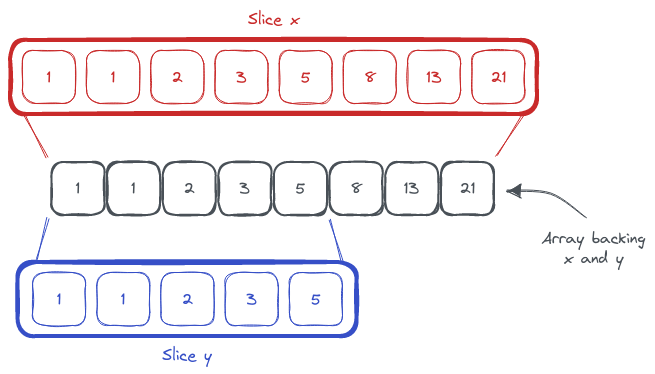# Go Tidbit: Filtering Slices In-place

March 6, 2023

At launch, Go did many things that felt out of place next to the other popular programming languages of the era. But now, those same language features feel so natural that I find it hard to think of a time without them.One such feature is the slice type. In Go, a slice is a view of an array. And naturally, you can have multiple slices backed by the same array:

 ``````1 2 3 4 5 `````` ``````x := []int{1, 1, 2, 3, 5, 8, 13, 21} y := x[:5] len(x) // == 8 len(y) // == 5 ``````

The length of the slice `x` is 8. The length of the slice `y` is 5. But both `x` and `y` are backed by the same array. If you were to change the value of `x`, the value of `y` would also change. Here `y` is a 5-element slice of the array backing `x`.

But you can also make a zero-element slice.

 ``````1 2 3 4 5 `````` ``````x := []int{1, 1, 2, 3, 5, 8, 13, 21} y := x[:0] len(x) // == 8 len(y) // == 0 ``````

At first, a zero-element slice may not feel very useful. You cannot access any element of the array through `y`. But, you can `append` to it.

 `````` 1 2 3 4 5 6 7 8 9 10 `````` ``````x := []int{1, 1, 2, 3, 5, 8, 13, 21} y := x[:0] len(y) // == 0 y = append(y, 99) len(y) // == 1 x // == 99 y // == 99 ``````

Now you can see where this is going and how you can filter slices without keeping two indexes like the old fashion way.

 ``````1 2 3 4 5 6 7 8 9 `````` ``````func FilterIntsEven(x []int) []int { r := x[:0] for _, v := range x { if v % 2 == 0 { r = append(r, v) } } return r } ``````

This function does not allocate a new array. The slice `r` uses the same array that backs `x`.

And, for those who like to use generics in Go:

 ``````1 2 3 4 5 6 7 8 9 `````` ``````func Filter[T any](x []T, f func(T) bool) []T { r := x[:0] for _, v := range x { if f(v) { r = append(r, v) } } return r } ``````

This post is 19th of my #100DaysToOffload challenge. Want to get involved? Find out more at 100daystooffload.com.